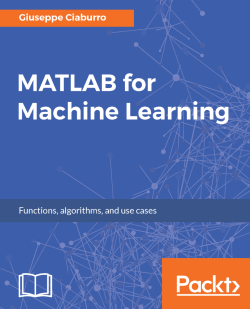MATLAB for Machine Learning5 (3 reviews total)
By Giuseppe Ciaburro
• \$5/mo for 5 months Subscribe Access now
• \$5.00 Was 39.99 eBook

• Constantly updated with 100+ new titles each month
• Breadth and depth in over 1,000+ technologies

MATLAB is the language of choice for many researchers and mathematics experts for machine learning. This book will help you build a foundation in machine learning using MATLAB for beginners.

You’ll start by getting your system ready with t he MATLAB environment for machine learning and you’ll see how to easily interact with the Matlab workspace. We’ll then move on to data cleansing, mining and analyzing various data types in machine learning and you’ll see how to display data values on a plot. Next, you’ll get to know about the different types of regression techniques and how to apply them to your data using the MATLAB functions.

You’ll understand the basic concepts of neural networks and perform data fitting, pattern recognition, and clustering analysis. Finally, you’ll explore feature selection and extraction techniques for dimensionality reduction for performance improvement.

At the end of the book, you will learn to put it all together into real-world cases covering major machine learning algorithms and be comfortable in performing machine learning with MATLAB.

Publication date:
August 2017
Publisher
Packt
Pages
382
ISBN
9781788398435

Chapter 1. Getting Started with MATLAB Machine Learning

Why is it so difficult for you to accept my orders if you're just a machine? Just a machine? That's like saying that you are just an ape. This is a short dialog between the leading actor and a robot, taken from the movie Automata. In this movie, the robots have two unalterable protocols; the first obliges them to preserve human life and the second limits them from repairing themselves. Why should humans limit the ability of robots to repair themselves? Because robots have a great capacity for self-learning that could lead them to take control of humans, over time maybe.

At least that is what happens in the movie.

But what do we really mean by self-learning? A machine has the ability to learn if it is able to improve its performance through its activities. Therefore, this ability can be used to help humans solve specific problems such as extracting knowledge from large amounts of data. In this chapter, we will be introduced to the basic concepts of machine learning, and then we will take a tour of the different types of algorithm. In addition, an introduction, some background information, and a basic knowledge of the MATLAB environment will be covered. Finally, we will explore the essential tools that MATLAB provides for understanding the amazing world of machine learning.In this chapter, we will cover the following topics:

• Discovering the machine learning capabilities in MATLAB for classification, regression, clustering, and deep learning, including apps for automated model training and code generation
• Taking a tour of the most popular machine learning algorithms to choose the right one for our needs
• Understanding the role of statistics and algebra in machine learning

At the end of the chapter, you will be able to recognize the different machine learning algorithms and the tools that MATLAB provides to handle them.

ABC of machine learning

Defining machine learning is not a simple matter; to do that, we can start from the definitions given by leading scientists in the field:

"Machine Learning: Field of study that gives computers the ability to learn without being explicitly programmed."                                                                                                 – Arthur L. Samuel(1959)

Otherwise, we can also provide a definition as:

"Learning denotes changes in the system that are adaptive in the sense that they enable the system to do the same task or tasks drawn from the same population more efficiently and more effectively the next time."                                                                                          – Herbert Alexander Simon (1984)

Finally, we can quote the following:

"A computer program is said to learn from experience E with respect to some class of tasks T and performance measure P, if its performance at tasks in T, as measured by P, improves with experience E".                                                                                                       – Tom M. Mitchell(1998)

In all cases, they refer to the ability to learn from experience without any outside help. Which is what we do humans in most cases. Why should it not be the same for machines?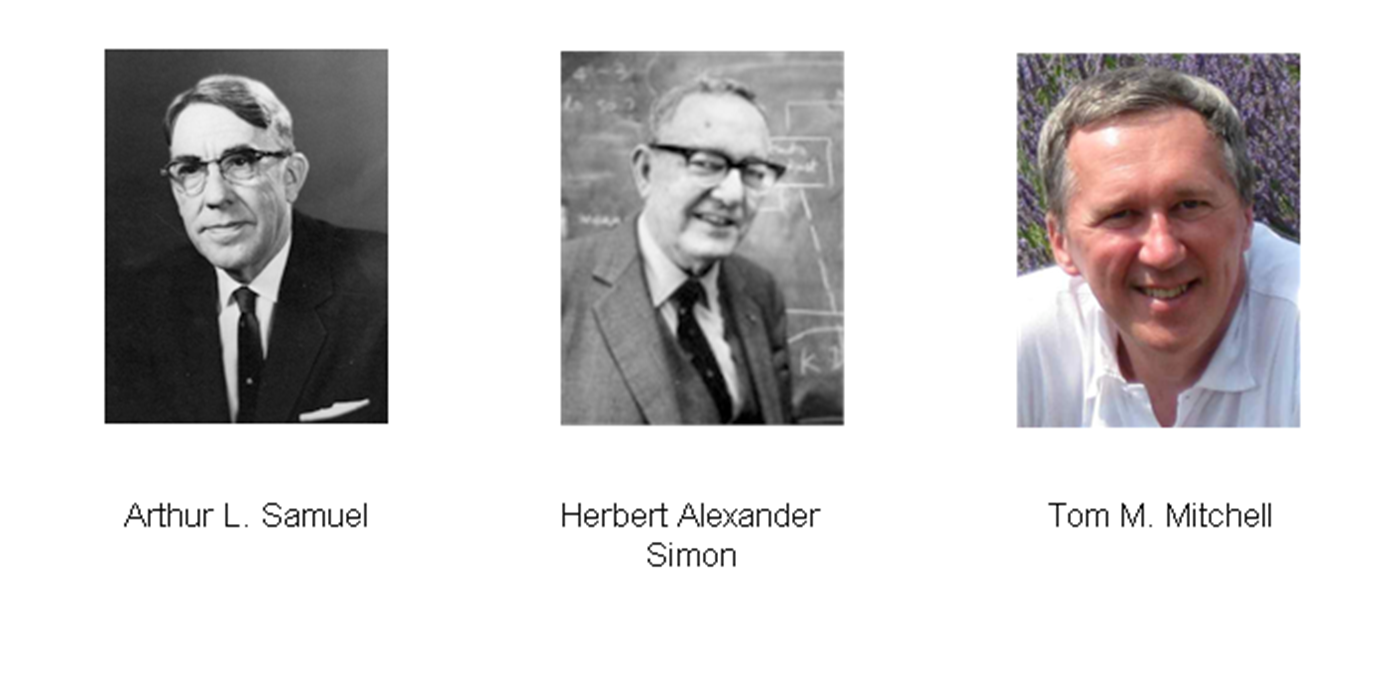Figure 1.1: The history of machine learning

Machine learning is a multidisciplinary field created by intersection and synergy between computer science, statistics, neurobiology, and control theory. Its emergence has played a key role in several fields and has fundamentally changed the vision of software programming. If the question before was, How to program a computer? now the question becomes, How will computers program themselves?

Thus, it is clear that machine learning is a basic method that allows a computer to have its own intelligence.

As it might be expected, machine learning interconnects and coexists with the study of, and research on, human learning. Like humans, whose brain and neurons are the foundation of insight, Artificial Neural Networks (ANNs) are the basis of any decision-making activity of the computer.

From a set of data, we can find a model that describes it by the use of machine learning. For example, we can identify a correspondence between input variables and output variables for a given system. One way to do this is to postulate the existence of some kind of mechanism for the parametric generation of data, which, however, does not know the exact values of the parameters. This process typically makes reference to statistical techniques such as Induction, Deduction, and Abduction, as shown in the following figure: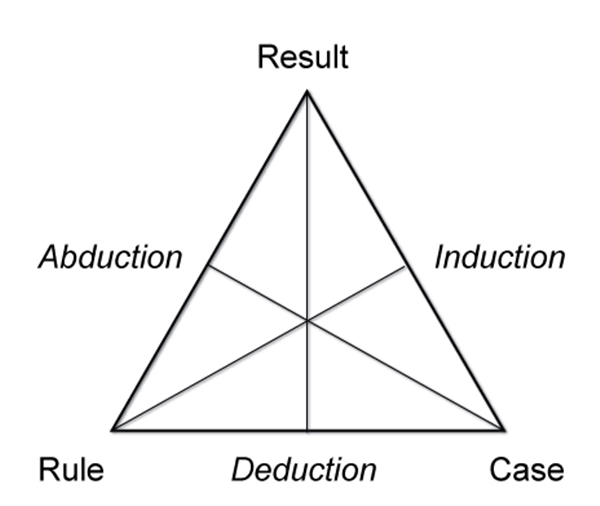Figure 1.2:  Peirce’s triangle - scheme of the relationship between reasoning patterns

The extraction of general laws from a set of observed data is called induction; it is opposed to deduction, in which, starting from general laws, we want to predict the value of a set of variables. Induction is the fundamental mechanism underlying the scientific method, in which we want to derive general laws (typically described in a mathematical language) starting from the observation of phenomena.

This observation includes the measurement of a set of variables and therefore the acquisition of data that describes the observed phenomena. Then, the resulting model can be used to make predictions on additional data. The overall process in which, starting from a set of observations, we want to make predictions for new situations is called inference.

Therefore, inductive learning starts from observations arising from the surrounding environment and generalizes obtaining knowledge that will be valid for not-yet-observed cases; at least we hope so.

We can distinguish two types of inductive learning:

• Learning by example: Knowledge gained by starting from a set of positive examples that are instances of the concept to be learned and negative examples that are non-instances of the concept.
• Learning regularity: This is not a concept to learn. The goal is to find regularity (common characteristics) in the instances provided.

The following figure shows the types of inductive learning:Figure 1.3: Types of inductive learning

A question arises spontaneously: Why do machine learning systems work while traditional algorithms fail? The reasons for the failure of traditional algorithms are numerous and typically due to the following:

• Difficulty in problem formalization: For example, each of us can recognize our friends from their voice. But probably none can describe a sequence of computational steps enabling them to recognize the speaker from the recorded sound.
• High number of variables at play: When considering the problem of recognizing characters from a document, specifying all parameters that are thought to be involved can be particularly complex. In addition, the same formalization applied in the same context but on a different idiom could prove inadequate.
• Lack of theory: Imagine you have to predict exactly the performance of financial markets in the absence of specific mathematical laws.
• Need for customization: The distinction between interesting and uninteresting features depends significantly on the perception of the individual user.

Here is a flowchart showing inductive and deductive learning:Figure 1.4: Inductive and deductive learning flowchart

Discover the different types of machine learning

The power of the machine learning is due to the quality of its algorithms, which have been improved and updated over the years; these are divided into several main types depending on the nature of the signal used for learning or the type of feedback adopted by the system. They are:

• Supervised learning: The algorithm generates a function that links input values ​​to a desired output through the observation of a set of examples in which each data input has its relative output data, and that is used to construct predictive models.
• Unsupervised learning : The algorithm tries to derive knowledge from a general input without the help of a set of pre-classified examples that are used to build descriptive models. A typical example of the application of these algorithms are search engines.
• Reinforcementlearning:  The algorithm is able to learn depending on the changes that occur in the environment in which it is performed. In fact, since every action has some effect on the environment concerned, the algorithm is driven by the same feedback environment. Some of these algorithms are used in speech or text recognition.

The following is a figure depicting the different types of machine learning algorithms: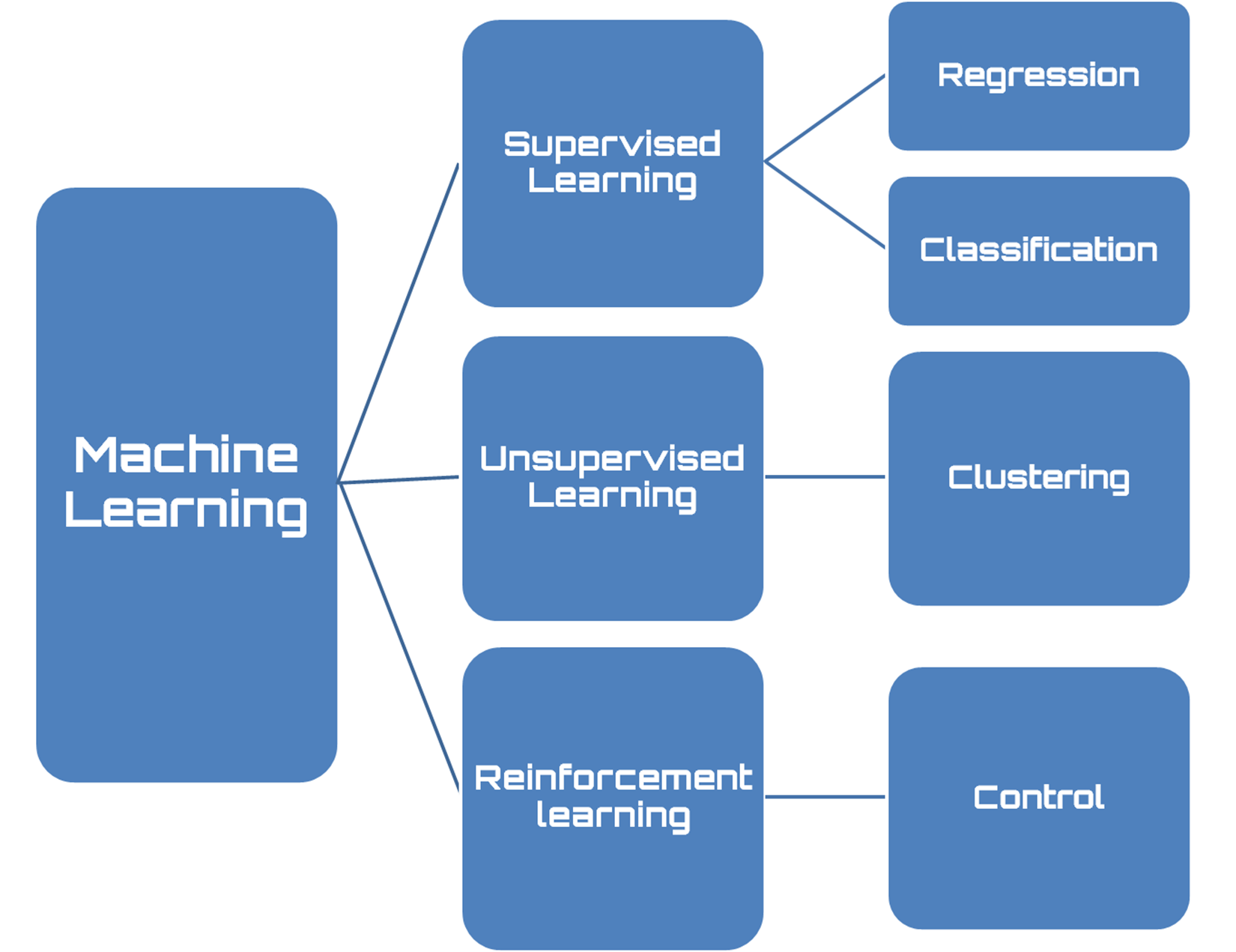Figure 1.5: Types of machine learning algorithms

Supervised learning

Supervised learning is a machine learning technique that aims to program a computer system so that it can resolve the relevant tasks automatically. To do this, the input data is included in a set I, (typically vectors). Then the set of output data is fixed as set O, and finally it defines a function f that associates each input with the correct answer. Such information is called a training set. This workflow is presented in the following figure: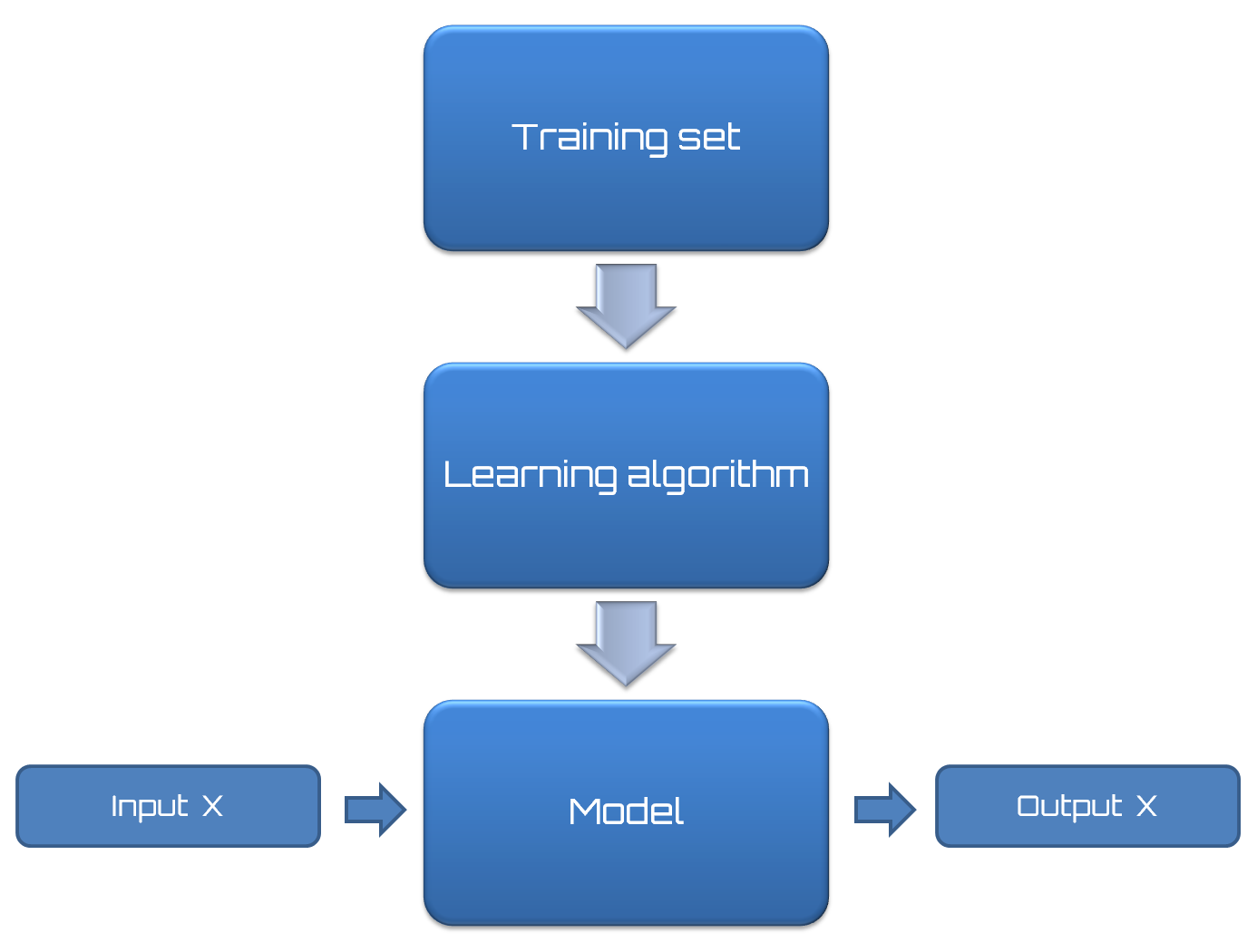Figure 1.6: Supervised learning workflow

All supervised learning algorithms are based on the following thesis: if an algorithm provides an adequate number of examples, it will be able to create a derived function B that will approximate the desired function A.

If the approximation of the desired function is adequate, when the input data is offered to the derived function, this function should be able to provide output responses similar to those provided by the desired function and then acceptable.These algorithms are based on the following concept: similar inputs correspond to similar outputs.Generally, in the real-world, this assumption is not valid; however, some situations exist in which it is acceptable. Clearly, the proper functioning of such algorithms depends significantly on the input data. If there are only a few training inputs, the algorithm might not have enough experience to provide a correct output. Conversely, many inputs may make it excessively slow since the derivative function generated by a large number of inputs could be very complicated.

Moreover, experience shows that this type of algorithm is very sensitive to noise; even a few pieces of incorrect data can make the entire system unreliable and lead to wrong decisions.

In supervised learning, it's possible to split problems based on the nature of the data. If the output value is categorical, such as membership/non-membership to a certain class, it is a classification problem. If the output is a continuous real value in a certain range, then it is a regression problem.

Unsupervised learning

The aim of unsupervised learning is to automatically extract information from databases. This process occurs without prior knowledge of the contents to be analyzed. Unlike supervised learning, there is no information on membership classes of the examples or generally on the output corresponding to a certain input. The goal is to get a model that is able to discover interesting properties: groups with similar characteristics (clustering) for instance. Search engines are an example of an application of these algorithms. Given one or more keywords, they are able to create a list of links related to our search.

The validity of these algorithms depends on the usefulness of the information they can extract from the databases. These algorithms work by comparing data and looking for similarities or differences. Available data concerns only the set of features that describe each example.

The following figure shows supervised learning and unsupervised learning examples: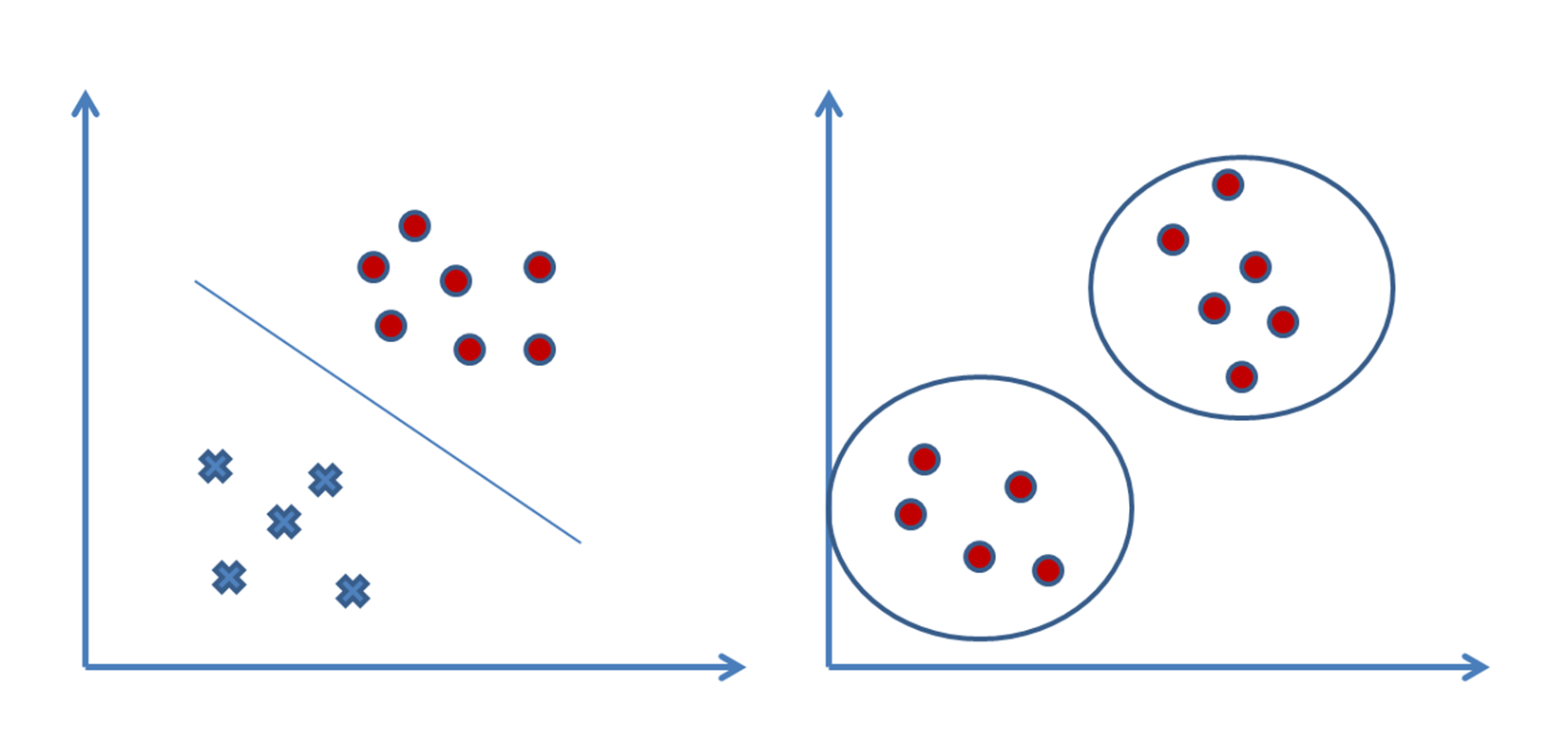Figure 1.7: Supervised learning versus unsupervised learning

They show great efficiency with elements of numeric type, but are much less accurate with non-numeric data. Generally, they work properly in the presence of data that contains an order or a clear grouping and is clearly identifiable.

Reinforcement learning

Reinforcement learning aims to create algorithms that can learn and adapt to environmental changes. This programming technique is based on the concept of receiving external stimuli depending on the algorithm choices. A correct choice will involve a premium while an incorrect choice will lead to a penalty. The goal of system is to achieve the best possible result, of course.

In supervised learning, there is a teacher that tells the system which is the correct output (learning with a teacher). This is not always possible. Often we have only qualitative information (sometimes binary, right/wrong, or success/failure).

The information available is called reinforcement signals. But the system does not give any information on how to update the agent's behavior (that is, weights). You cannot define a cost function or a gradient. The goal of the system is to create the smart agents that have a machinery able to learn from their experience.

This flowchart shows reinforcement learning: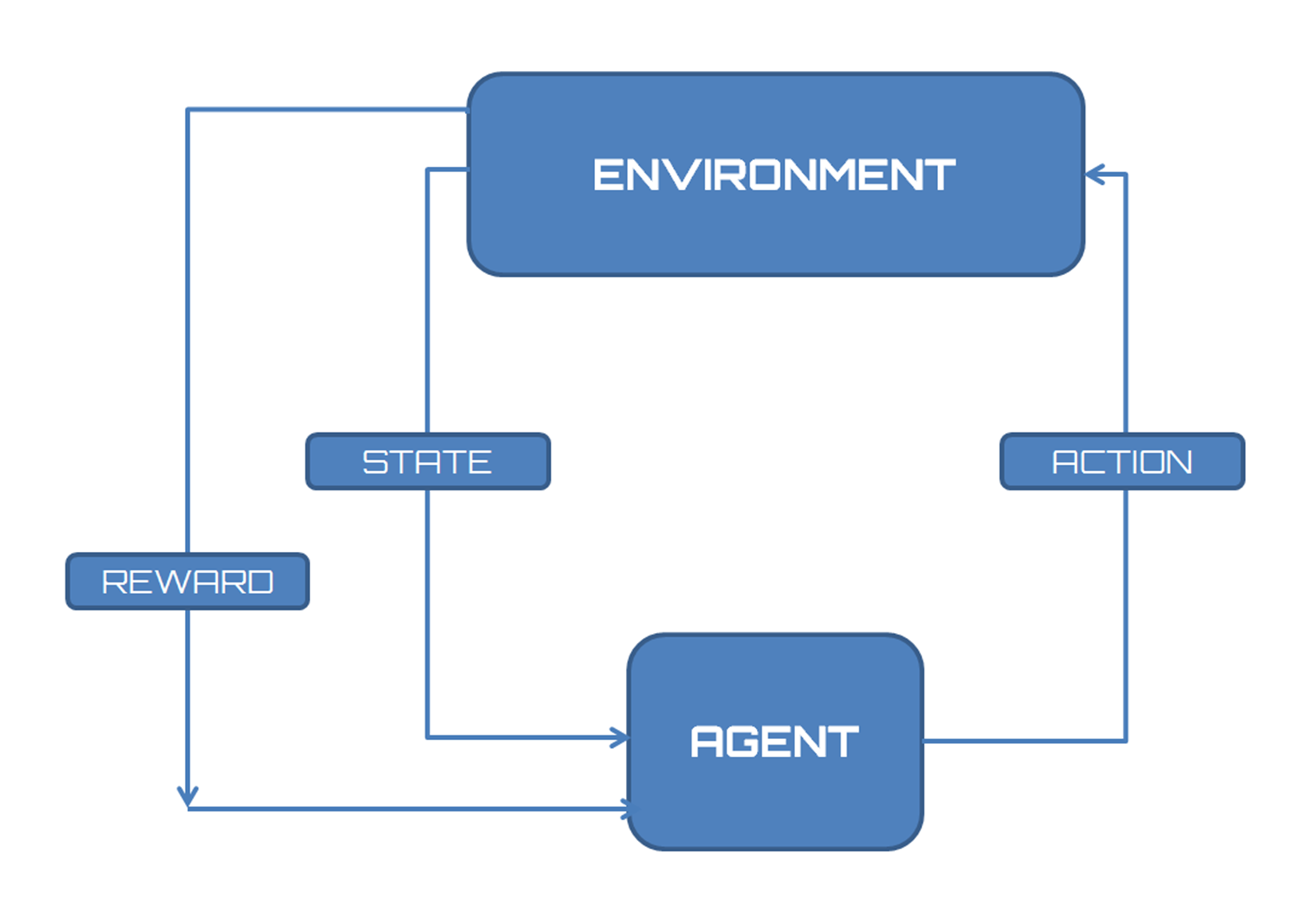Figure 1.8: How to reinforcement learning interact with the environment

Choosing the right algorithm

In the previous section, we learned the difference between various types of machine learning algorithms. So, we understood the basic principles that underlie the different techniques. Now it's time to ask ourselves the following question: What is the right algorithm for my needs?Unfortunately there is no common answer for everyone, except the more generic: It depends. But what does it depend on? It mainly depends on the data available to us: the size, quality, and nature of the data. It depends on what we want to do with the answer. It depends on how the algorithm has been expressed in instructions for the computer. It depends on how much time we have. There is no best method or one-size-fits-all. The only way to be sure that the algorithm chosen is the right one is to try it.

However, to understand what is most suitable for our needs, we can perform a preliminary analysis. Beginning from what we have (data), what tools we have available (algorithms), and what objectives we set for ourselves (the results), we can obtain useful information on the road ahead.

If we start from what we have (data), it is a classification problem, and two options are available:

• Classify based on input: We have a supervised learning problem if we can label the input data. If we cannot label the input data but want to find the structure of the system, then it is unsupervised. Finally, if our goal is to optimize an objective function by interacting with the environment, it is a reinforcement learning problem.
• Classify based on output: If our model output is a number, we have to deal with a regression problem. But it is a classification problem if the output of the model is a class. Finally, we have a clustering problem if the output of the model is a set of input groups.

The following is a figure that shows two options available in the classification problem: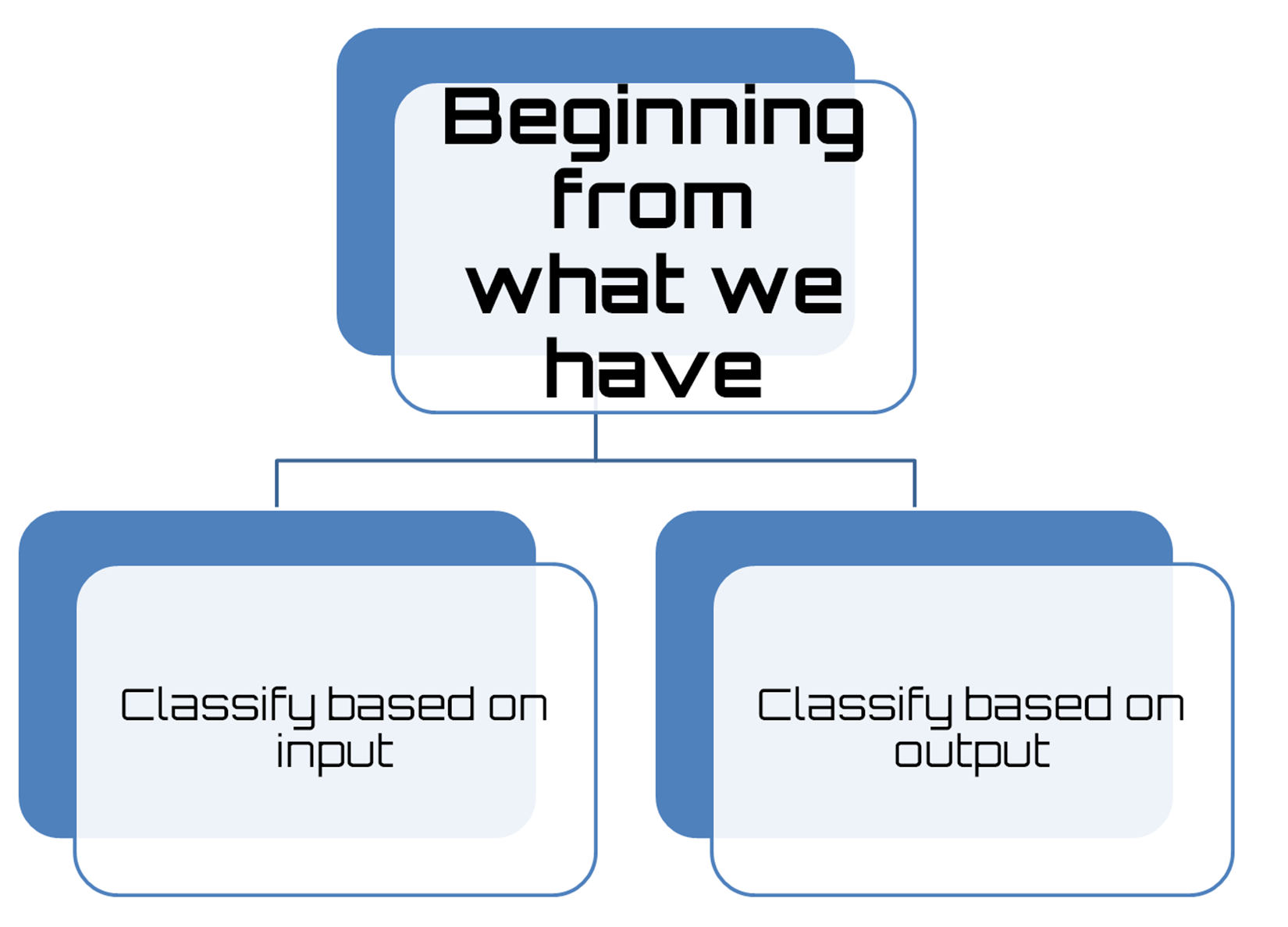Figure 1.9: Preliminary analysis

After classifying the problem, we can analyze the tools available to solve the specific problem. Thus, we can identify the algorithms that are applicable and focus our study on the methods to be implemented to apply these tools to our problem.

Having identified the tools, we need to evaluate their performance. To do this, we simply apply the selected algorithms on the datasets at our disposal. Subsequently, on the basis of a series of carefully selected evaluation criteria, we carry out a comparison of the performance of each algorithm.

How to build machine learning models step by step

Finally, the algorithm to apply to our data is chosen; it is now time to get down to work without delay. Before you tackle such a job, it is appropriate to devote some time to the workflow setting. When developing an application that uses machine learning, we will follow a procedure characterized by the following steps:

1. Collect the data: Everything starts from the data, no doubt about it; but one might wonder where so much data comes from. In practice, it is collected through lengthy procedures that may, for example, derive from measurement campaigns or face-to-face interviews. In all cases, the data is collected in a database so that it can then be analyzed to derive knowledge.

Note

If we do not have specific requirements, and to save time and effort, we can use publicly available data. In this regard, a large collection of data is available in the UCI Machine Learning Repository at the following link: http://archive.ics.uci.edu/ml.

The following figure shows how to build machine learning models step by step: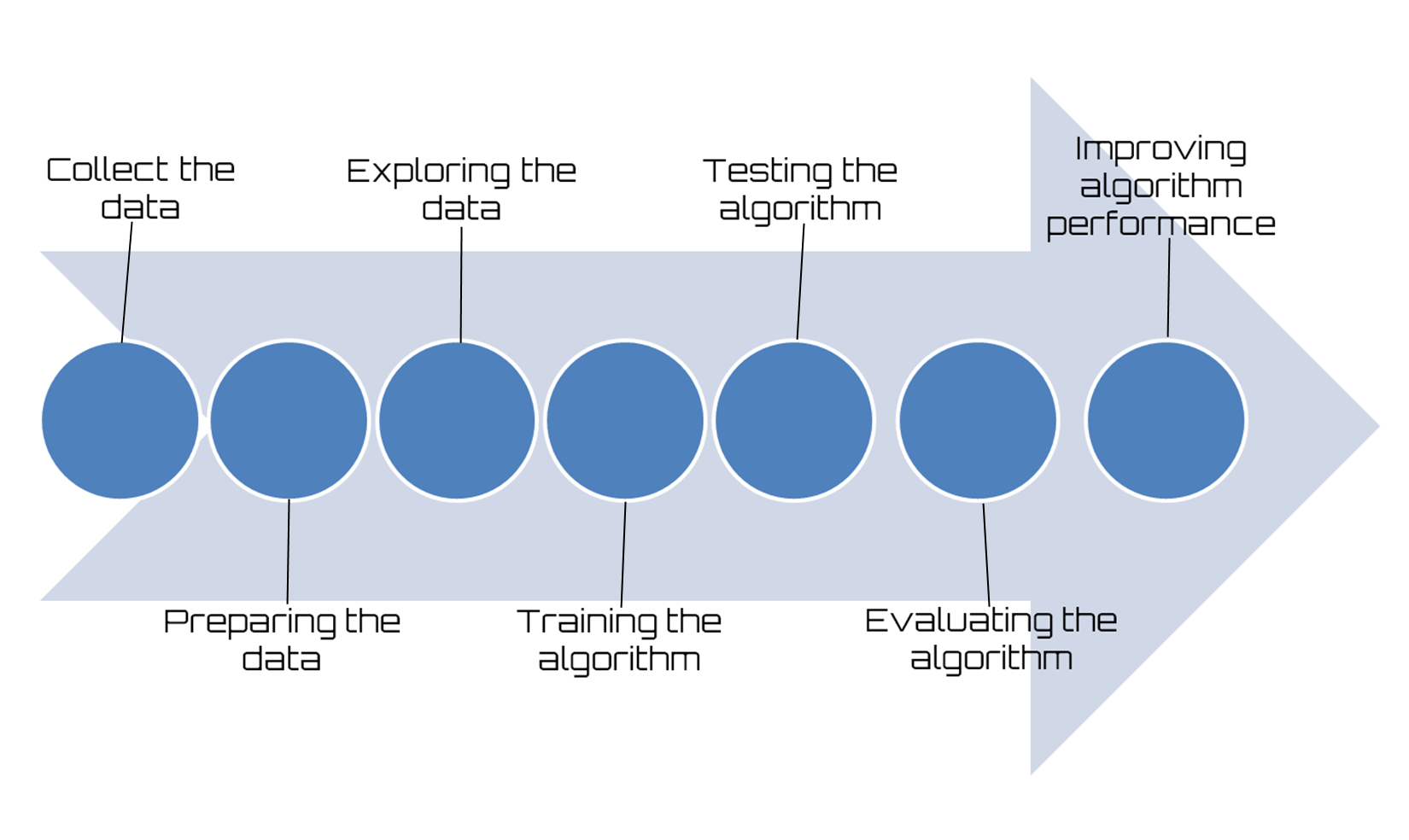Figure 1.10: Machine learning workflow

1. Preparing the data: We have collected the data; now we have to prepare it for the next step. Once we have this data, we must make sure it is in a format usable by the algorithm we want to use. To do this, you may need to do some formatting. Recall that some algorithms need data in an integer format, whereas others require data in the form of strings, and finally others need to be in a special format. We will get to this later, but the specific formatting is usually simple compared to data collection.
2. Exploring the data: At this point, we can look at data to verify that it is actually working and we do not have a bunch of empty values. In this step, through the use of plots, we can recognize patterns or whether there are some data points that are vastly different from the rest of the set. Plotting data in one, two, or three dimensions can also help.
3. Training the algorithm: Now let's get serious. In this step, the machine learning begins to work with the definition of the model and the next training. The model starts to extract knowledge from large amounts of data that we had available, and that nothing has been explained so far. For unsupervised learning, there’s no training step because you don’t have a target value.
4. Testing the algorithm: In this step, we use the information learned in the previous step to see if the model actually works. The evaluation of an algorithm is for seeing how well the model approximates the real system. In the case of supervised learning, we have some known values that we can use to evaluate the algorithm. In unsupervised learning, we may need to use some other metrics to evaluate success. In both cases, if we are not satisfied, we can return to the previous steps, change some things, and retry the test.
5. Evaluating the algorithm: We have reached the point where we can apply what has been done so far. We can assess the approximation ability of the model by applying it to real data. The model, preventively trained and tested, is then valued in this phase.
6. Improving algorithm performance: Finally we can focus on the finishing steps. We've verified that the model works, we have evaluated the performance, and now we are ready to analyze the whole process to identify any possible room for improvement.

Introducing machine learning with MATLAB

So far, we have learned what machine learning algorithms do; we have also understood how to recognize the different types, how to locate the right solution for our needs, and finally how to set a proper workflow. It's time to learn how to do all this in the MATLAB environment.

Solving machine learning problems becomes extremely easy with the use of the tools available in the MATLAB environment. This is because MATLAB is a strong environment for interactive exploration. It has numerous algorithms and apps to help you get started using machine learning techniques. Some examples include:

• Clustering, classification, and regression algorithms
• Neural network app, curve fitting app, and Classification Learner app

MATLAB is a software platform optimized for solving scientific problems and design. In MATLAB, calculation, visualization, and programming are integrated in an easy-to-use environment, where problems and solutions are expressed in familiar mathematical notation.

The name MATLAB is an acronym of the term matrix laboratory. MATLAB was originally written to provide easy access to software of matrices; then it evolved in the years to come, thanks to numerous user inputs. The MATLAB programming language is based on matrices that represent the most natural way to express computational mathematics. Its desktop environment invites experimentation, exploration, and discovery. The integrated graphics are easy to view and provide an in-depth understanding of the data.

The MATLAB desktop is shown in the following screenshot: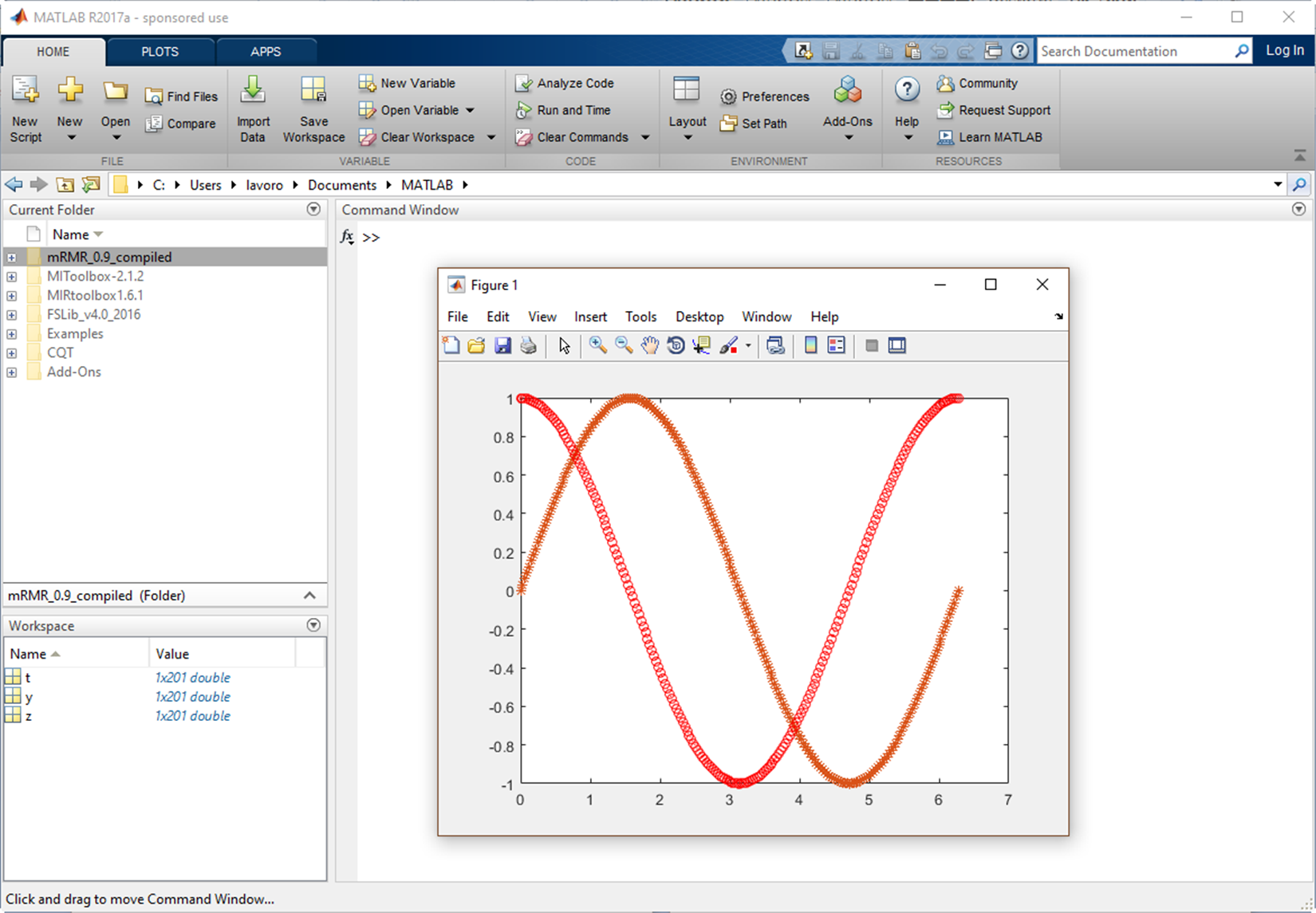Figure 1.11: MATLAB desktop

MATLAB is also characterized by the presence of specific solutions to application problems called toolboxes. Very useful for most users, MATLAB toolboxes represent solutions for many practical problems and provide the basis for applying these instruments to the specialized technology. These toolboxes are collections of MATLAB functions (referred to as M-files) that extend the MATLAB environment in order to solve particular classes of problems.

MATLAB has two specific toolboxes for processing machine learning problems. They are the Statistics and Machine Learning Toolbox and Neural Network Toolbox. While the first solves machine learning problems through statistical techniques and algorithms most widely used in this field, the second is specific to ANNs. In the following sections, we will analyze in detail the features of these tools.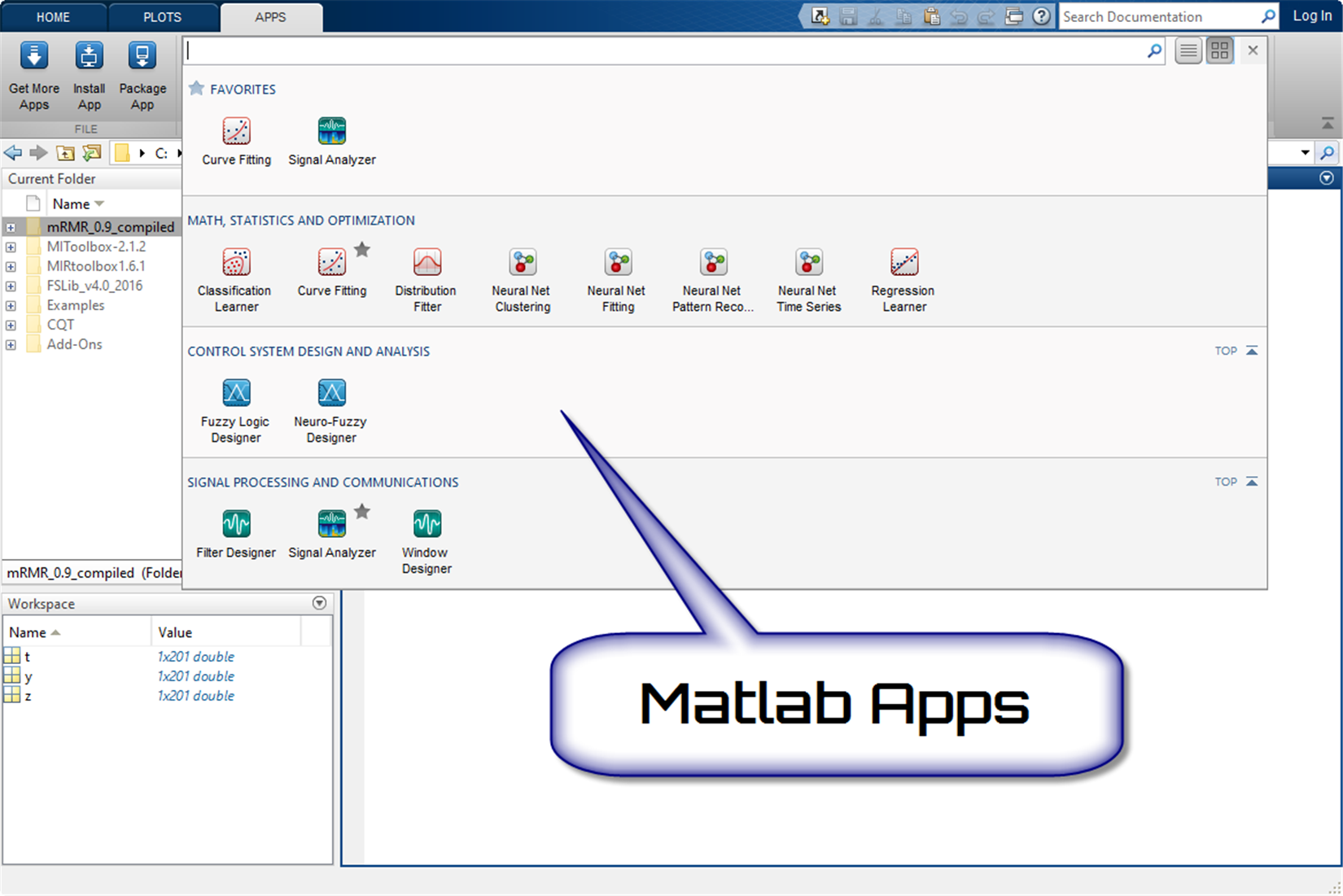Figure 1.12: Some apps available in MATLAB

System requirements and platform availability

To be used efficiently, all computer software needs certain hardware components or other software resources to be present on a computer. Thus, MATLAB requires specific hardware to be installed and working properly on our computer. Fortunately, we can start from the assumption that MATLAB is available for all popular software platforms in both professional and student editions. In fact, it is available for the Windows, macOS, and Linux platforms. But we can rest assured that what MATLAB requires is widely supported by new computers.

So the hardware requirements for Windows are:

• Operating systems: Windows 10, Windows 8.1, Windows 8, Windows 7 Service Pack 1, Windows Server 2016, Windows Server 2012 R2, Windows Server 2012, and Windows Server 2008 R2 Service Pack 1.
• Processors: Any Intel or AMD x86-64 processor; AVX2 instruction set support is recommended; with Polyspace, 4-core is recommended.
• Disk Space: 2 GB for MATLAB only, 4–6 GB for a typical installation.
• RAM: 2 GB; with Simulink, 4 GB is required; with Polyspace, 4 GB per core is recommended.
• Graphics: No specific graphics card is required. A hardware-accelerated graphics card supporting OpenGL 3.3 with 1 GB GPU memory is recommended.

Note

To discover the hardware requirements for other platforms, visit the manufacturer's website at the following link: https://www.mathworks.com/support/sysreq.html.

In the following screenshot, the hardware requirements for Windows are listed: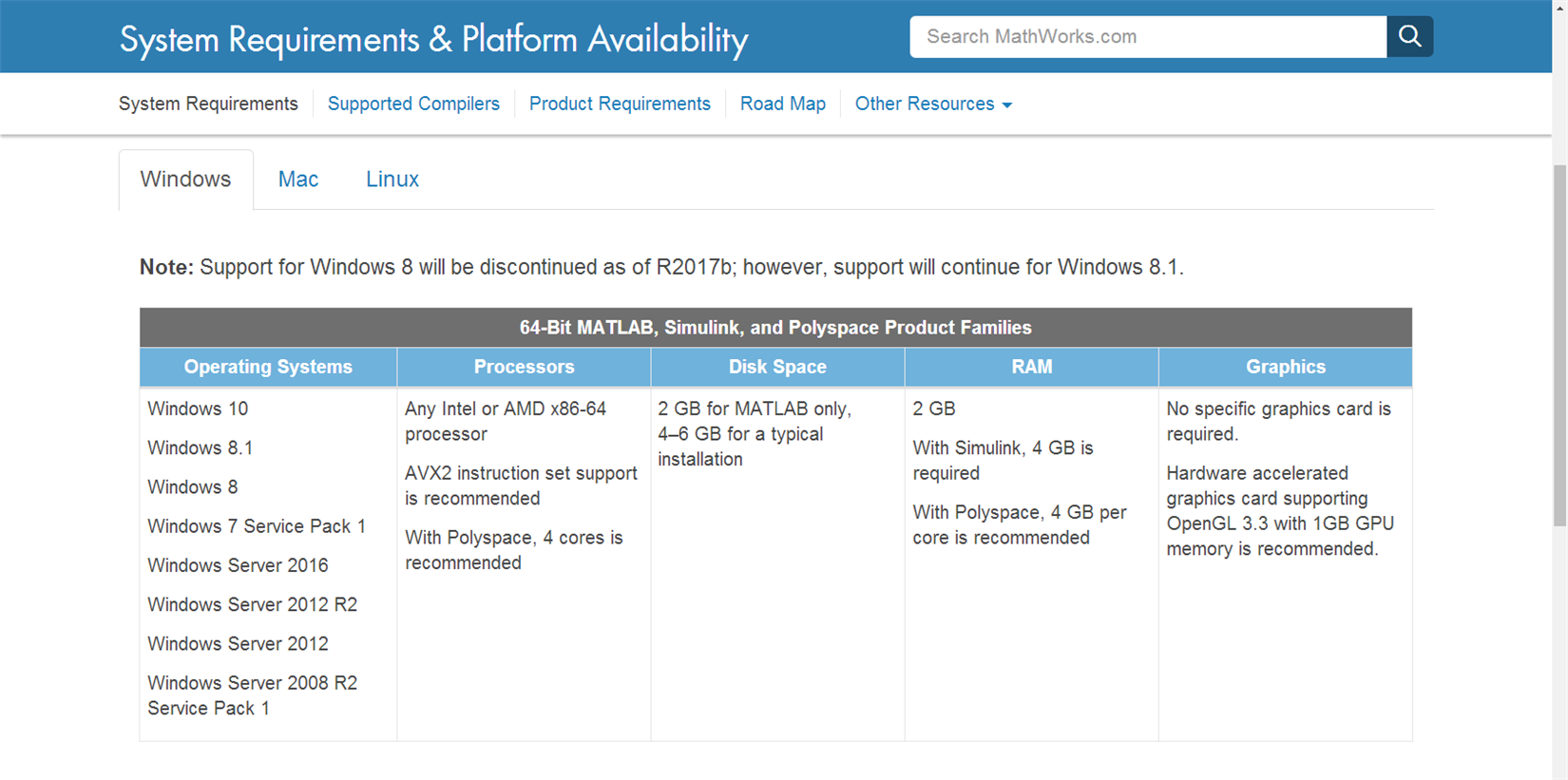Figure 1.13: Windows hardware requirements for MATLAB.

Once we have the MathWorks installer, to start the installation, we run this file and select the products we want to use. To run the installer, we need the following:

• Consider disabling anti-virus software and Internet security applications on your system during installation. These applications can slow down the installation process or cause it to appear unresponsive.

Later we should simply follow the usual software installation procedure. In the end, we will get a version of MATLAB ready for use.

Statistics and Machine Learning Toolbox

The Statistics and Machine Learning Toolbox contains all the tools necessary to extract knowledge from large datasets. It provides functions and apps to analyze, describe, and model data. Starting exploratory data analysis becomes a breeze with the descriptive statistics and graphs contained in the toolbox. Furthermore, fitting probability distributions to data, generating random numbers, and performing hypothesis tests will be extremely easy. Finally through the regression and classification algorithms, we can draw inferences from data and build predictive models.

For data mining, the Statistics and Machine Learning Toolbox offers feature selection, stepwise regression, Principal Component Analysis (PCA), regularization, and other dimensionality reduction methods that allow the identification of variables or functions that impact your model.

In this toolbox are developed supervised and unsupervised machine learning algorithms, including Support Vector Machines (SVMs), decision trees, k-Nearest Neighbor (KNN), k-means, k-medoids, hierarchical clustering, Gaussian Mixture Models (GMM), and hidden Markov models (HMM). Through the use of such algorithms, calculations on datasets that are too large to be stored in memory can be correctly executed. In the following screenshot, product capabilities of the Statistics and Machine Learning Toolbox are shown, extracted from the MathWorks site: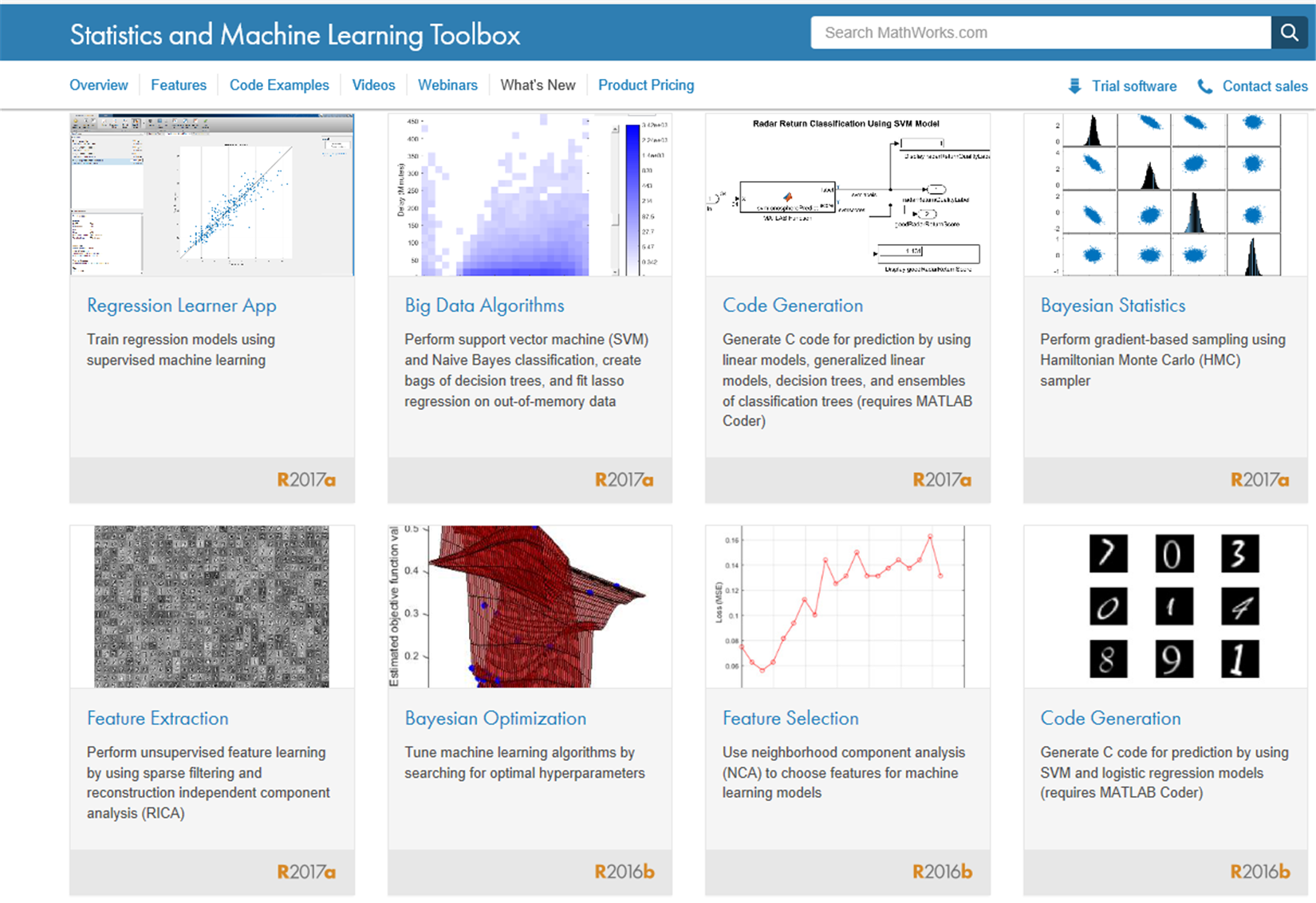Figure 1.14: Product capabilities of the Statistics and Machine Learning Toolbox

Here is a descriptive list of the key features of this tool; you will find the main topics of the machine learning field:

• Regression techniques, including linear, generalized linear, nonlinear, robust, regularized, ANOVA, repeated measures, and mixed-effects models
• Big data algorithms for dimension reduction, descriptive statistics, k-means clustering, linear regression, logistic regression, and discriminant analysis
• Univariate and multivariate probability distributions, random and quasi-random number generators, and Markov chain samplers
• Hypothesis tests for distributions, dispersion, and location; Design of Experiments (DOE) techniques for optimal, factorial, and response surface designs
• Classification Learner app and algorithms for supervised machine learning, including SVMs, boosted and bagged decision trees, KNN, Naive Bayes, discriminant analysis, and Gaussian process regression
• Unsupervised machine learning algorithms, including k-means, k-medoids, hierarchical clustering, Gaussian mixtures, and HMMs
• Bayesian optimization for tuning machine learning algorithms by searching for optimal hyperparameters

The following are the product resources of the Statistics and Machine Learning Toolbox: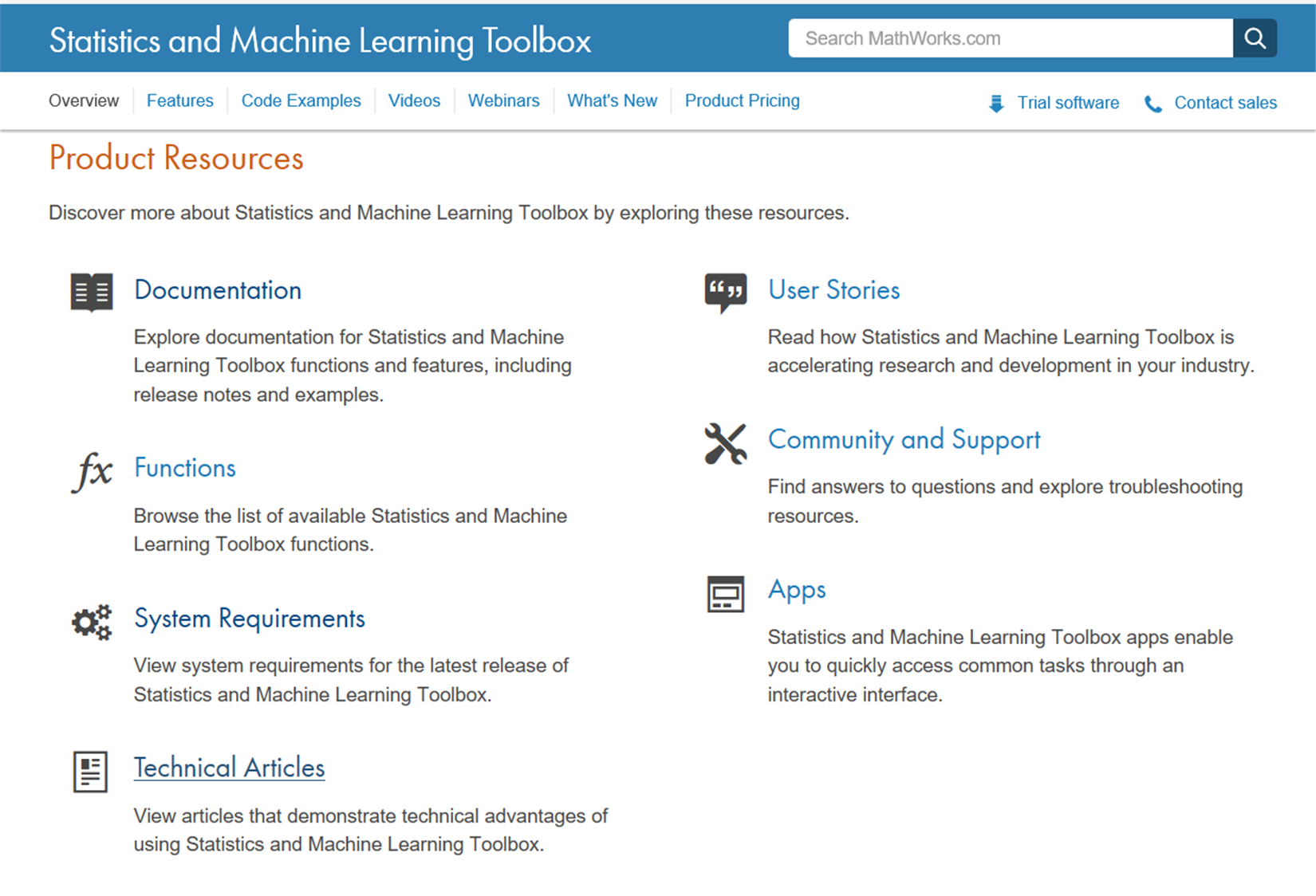Figure 1.15: Product resources of the Statistics and Machine Learning Toolbox

Note

For a more comprehensive overview of the Statistics and Machine Learning Toolbox's capabilities, you can connect to the manufacturer's website at the following link:  https://www.mathworks.com/products/statistics.html.

Datatypes

Before we start working with the Statistics and Machine Learning Toolbox, we should learn how to format the data to be processed by MATLAB. The Statistics and Machine Learning Toolbox supports only some specific datatypes for input arguments. MATLAB might return an error or unexpected results if we specify data in an unsupported type.

Supported datatypes

The datatypes supported are as follow:

• Numeric scalars, vectors, matrices, or arrays having single or double precision entries. These data forms have the single or double datatype.
• Cell arrays of character vectors; character, logical, or categorical arrays; or numeric vectors for categorical variables representing grouping data. These data forms have the cellstr, char, logical, categorical, and single or double datatype, respectively.
• Some functions support tabular arrays for heterogeneous. The table datatype contains variables of any of the datatypes previously listed.
• Some functions accept gpuArray input arguments so that they execute on the GPU.

Unsupported datatypes

These are as follows:

• Complex numbers.
• Custom numeric datatypes, for example, a variable that is double precision and an object.
• Signed or unsigned numeric integers for non-grouping data, for example, unint8 and int16.
• Sparse matrices, for example, matrix ONE such that issparse(ONE) returns 1. To use data that is of datatype sparse, recast the data to a matrix using full.

What can you do with the Statistics and Machine Learning Toolbox?

In the previous section, we analyzed the main features of the Statistics and Machine Learning Toolbox and how to format the data for following processes. It is now legitimate to ask: What can we really do with all the functions that MATLAB makes available to us? In this section, we will see a series of real case solutions through the use of such tools. Let us see some applications.

Data mining and data visualization

Data mining is the process of finding correlations among several fields of large relational databases. Through this process, data is analyzed from different perspectives and summarized into useful information. This information will then be used to adopt the necessary strategies to solve a problem.

MATLAB has several tools that allow us to perform a data mining analysis. In particular, the Statistics and Machine Learning Toolbox presents many techniques that give us the opportunity to obtain useful information from data. Good examples of these tools are:

• Statistical plotting with interactive graphics
• Descriptive statistics for large datasets

An example of visualizing multivariate data is shown in the following figure: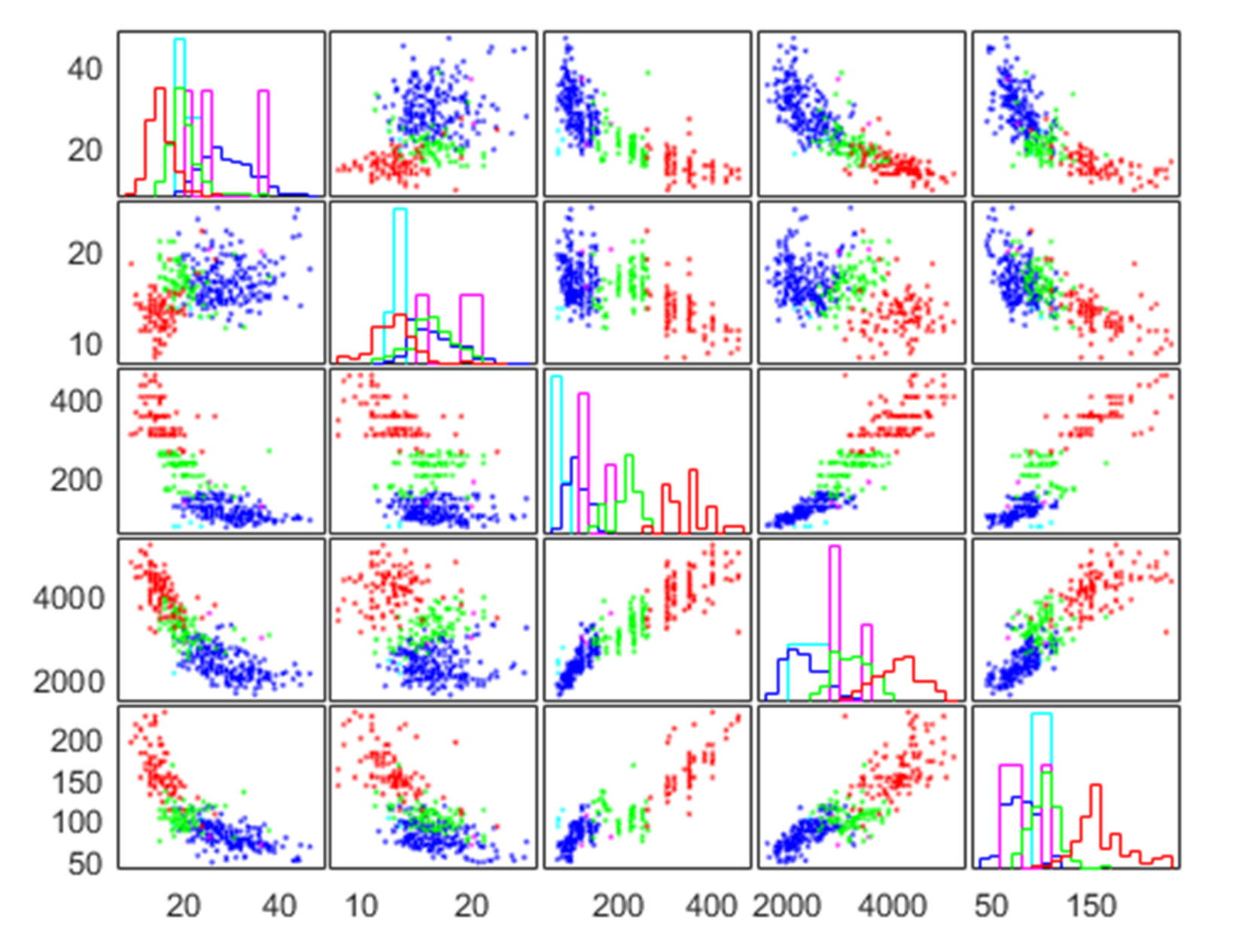Figure 1.16: Visualizing multivariate data

For example, we can start our analysis from visual exploration of data through a statistical plotting with interactive graphics. In this regard, MATLAB has many graphs and charts ready for use. In addition, the Statistics and Machine Learning Toolbox augments MATLAB plot types with probability plots, box plots, histograms, scatter histograms, 3D histograms, control charts, and quantile-quantile plots. For multivariate analysis, dendrograms, biplots, parallel coordinate charts, and Andrews plots are included in the toolbox.

In some cases, we must visualize multivariate data. Many statistical analyses require only two variables: A predictor variable (independent variable) and a response variable (dependent variable). The relationships between the two types of variables is easy to visualize using 2D scatter plots, bivariate histograms, boxplots, and so on. Similarly it is possible to extend the analysis to trivariate data and display it with 3D scatter plots, or 2D scatter plots with a third variable encoded. However, many datasets involve a larger number of variables, making direct visualization more difficult. In MATLAB, it's possible to visualize multivariate data using various statistical plots, through the Statistics and Machine Learning Toolbox (Figure 1.16).

Finally we can extract useful information using a descriptive statistic. A descriptive statistic identifies a set of techniques and tools aimed at fulfilling one of the top priorities of the statistic: describe, represent, and summarize the observed data to analyze a certain phenomenon. The Statistics and Machine Learning Toolbox includes functions for calculating:

• Measures of central tendency, including average, median, and various means
• Measures of dispersion, including range, variance, standard deviation, and mean or median absolute deviation
• Linear and rank correlation
• Results based on data with missing values
• Percentile and quartile estimates
• Density estimates using a kernel-smoothing function

Regression analysis

Regression analysis is a technique used to analyze a series of data that consists of a dependent variable and one or more independent variables. The purpose is to estimate a possible functional relationship between the dependent variable and the independent variables. Using this technique, we can build a model in which a continuous response variable is a function of one or more predictors.

In the Statistics and Machine Learning Toolbox, there are a variety of regression algorithms, including:

• Linear regression
• Nonlinear regression
• Generalized linear models
• Mixed-effects models

A scatter plot of the linear regression model is shown in the following figure.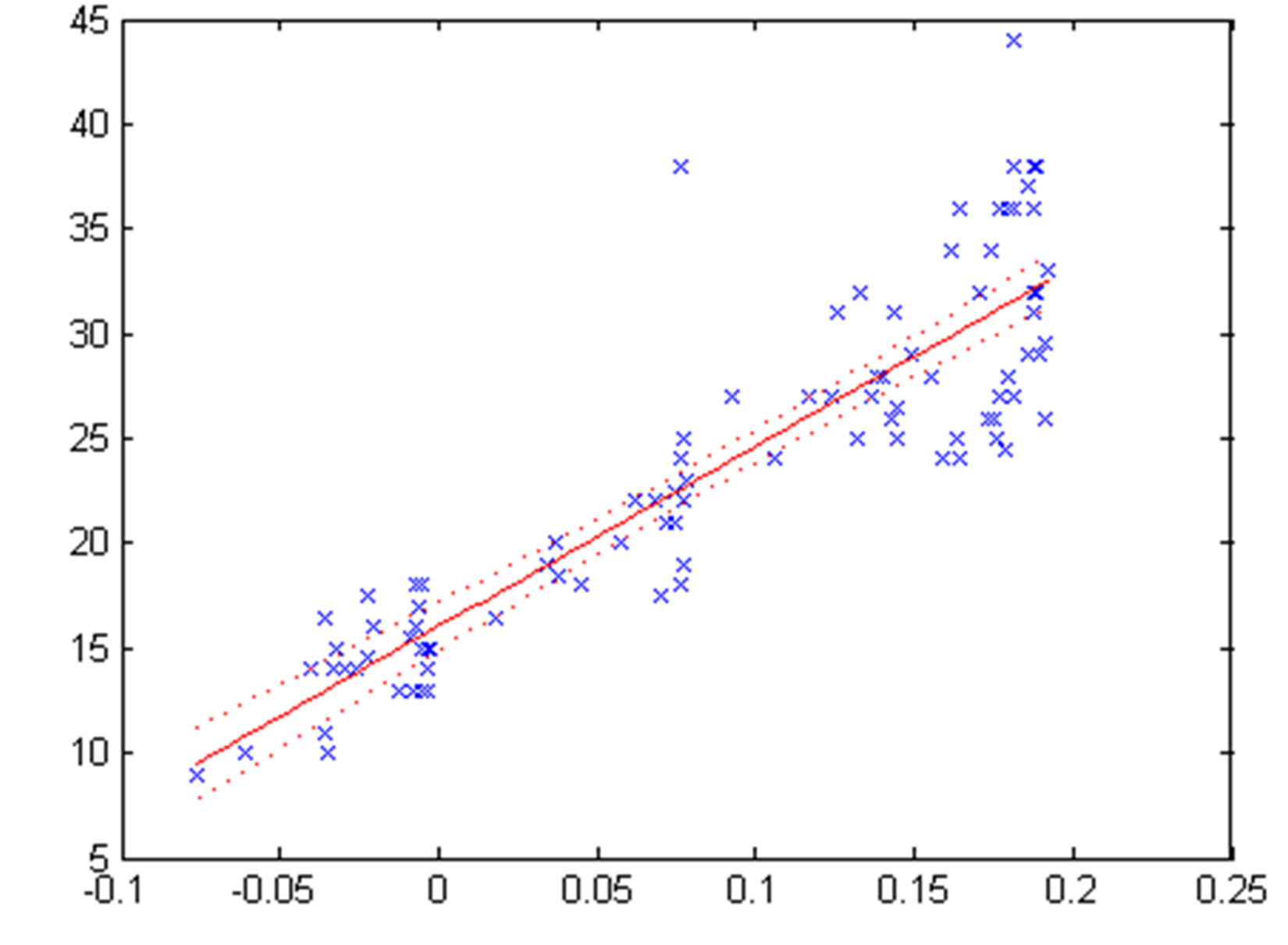Figure 1.17: Scatter plot of linear regression model

To study the relationship between two variables, a scatter plot is useful, in which we show the values of the independent variable X on the horizontal axis and the values of the dependent variable Y on the vertical axis. Using a regression model, we can express the relationship between two variables with functions that are more or less complex. Simple linear regression is suitable when the values of X and Y are distributed along a straight line in the scatter plot (Figure 1.17).

Classification

Classification models are supervised learning methods and are aimed at predicting a categorical target. From a set of observations for which the class is known, a model that allows us to make predictions is generated.

The Statistics and Machine Learning Toolbox offers apps and functions that cover a variety of parametric and non-parametric classification algorithms, such as:

• Logistic regression
• Boosted and bagged decision trees, including AdaBoost, LogitBoost, GentleBoost, and RobustBoost
• Naive Bayes classification
• KNN classification
• Discriminant analysis (linear and quadratic)
• SVM (binary and multiclass classification)

The Classification Learner app is a very useful tool that executes more request activities such as interactively explore data, select features, specify cross-validation schemes, train models, and assess results. We can use it to perform common tasks such as:

• Importing data and specifying cross-validation schemes
• Exploring data and selecting features
• Training models using several classification algorithms
• Comparing and assessing models
• Sharing trained models for use in applications such as computer vision and signal processing

Using the Classification Learner app, we can choose between various algorithms to train and validate classification models. After the training, compare the models' validation errors and choose the best one on the basis of results.

The following figure shows the Classification Learner app: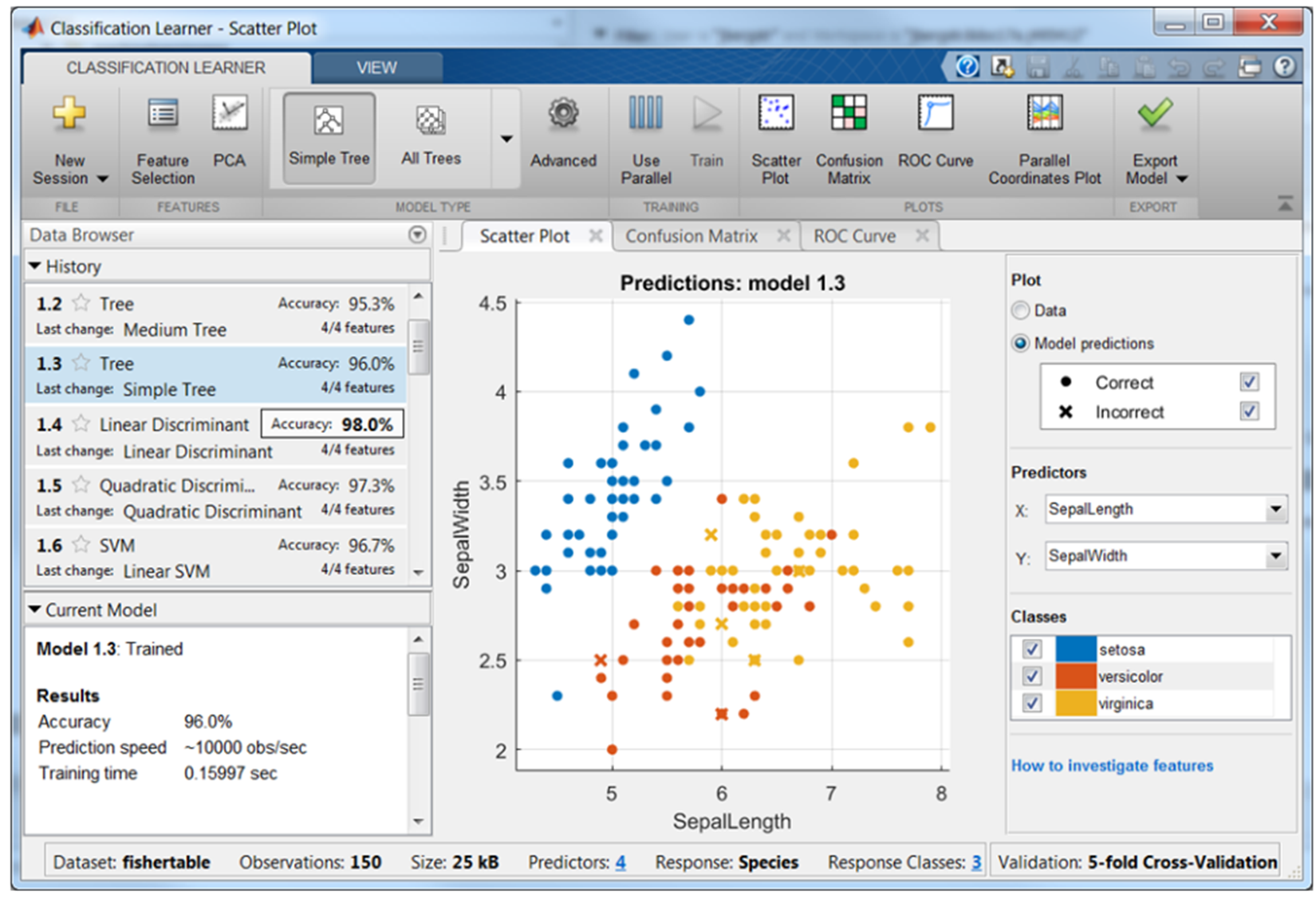Figure 1.18: The Classification Learner with a history list containing various classifier types

Cluster analysis

Cluster analysis is a multivariate analysis technique through which it is possible to group the statistical units so as to minimize the logic distance of each group and the logic distance between the groups. The logic distance is quantified by means of measures of similarity/dissimilarity between the defined statistical units.

The Statistics and Machine Learning Toolbox provides several algorithms to carry out cluster analysis. Available algorithms include:

• k-means
• k-medoids
• Hierarchical clustering
• GMM
• HMM

When the number of clusters is unknown, we can use cluster evaluation techniques to determine the number of clusters present in the data based on a specified metric.

A typical cluster analysis result is shown in the following figure: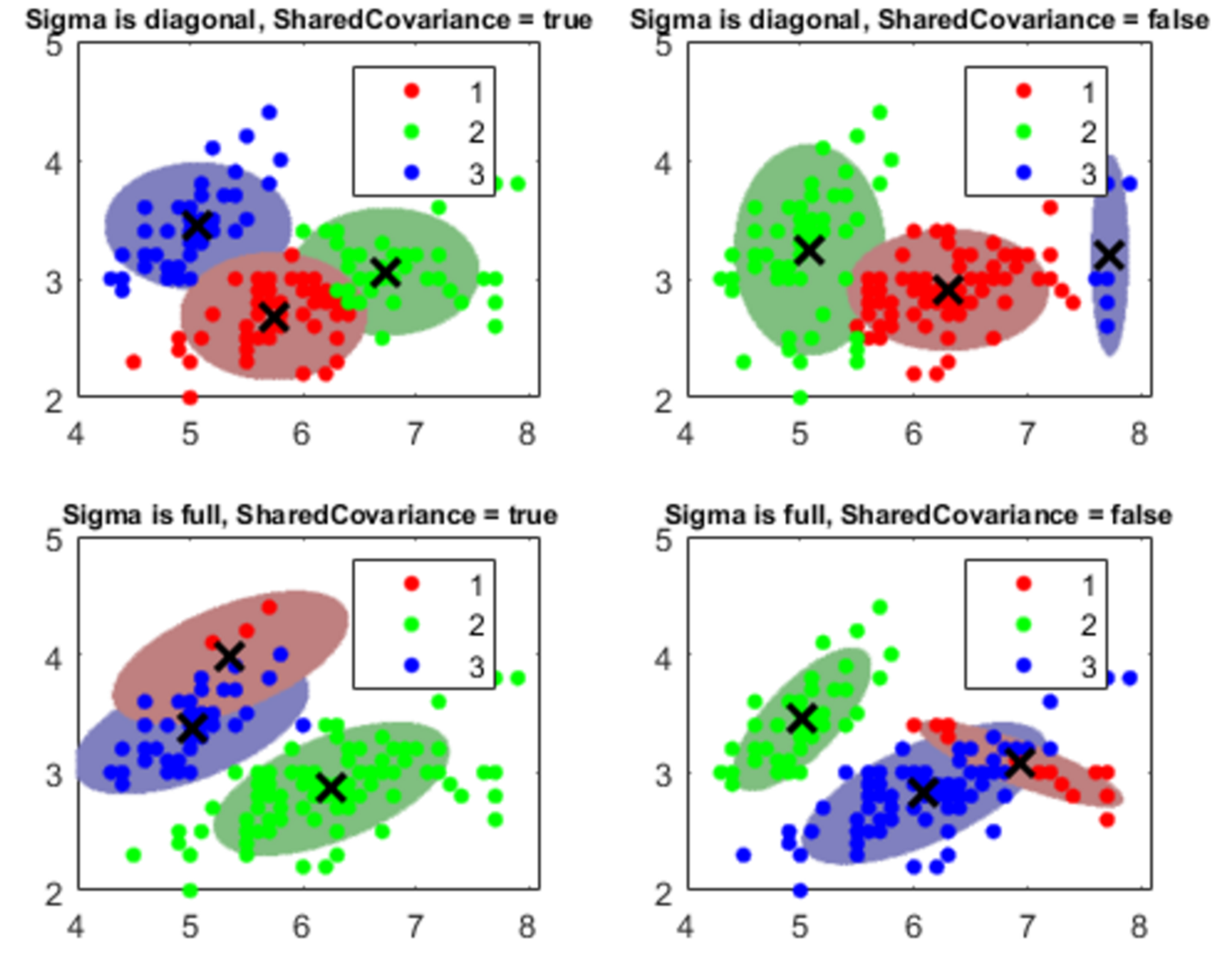Figure 1.19: A cluster analysis example

In addition, the Statistics and Machine Learning Toolbox allows viewing clusters by creating a dendrogram plot to display a hierarchical binary cluster tree. Then, we optimize the leaf order to maximize the sum of the similarities between adjacent leaves. Finally, for grouped data with multiple measurements for each group, we create a dendrogram plot based on the group means computed using a multivariate analysis of variance.

Dimensionality reduction

Dimensionality reduction is the process of converting a set of data with many variables into data with lesser dimensions but ensuring similar information. It can help improve model accuracy and performance, improve interpretability, and prevent overfitting. The Statistics and Machine Learning Toolbox includes many algorithms and functions for reducing the dimensionality of our datasets. It can be divided into feature selection and feature extraction. Feature selection approaches try to find a subset of the original variables. Feature extraction reduces the dimensionality in the data by transforming data into new features.

As already mentioned, feature selection finds only the subset of measured features (predictor variables) that give the best predictive performance in modeling the data. The Statistics and Machine Learning Toolbox includes many feature selection methods, as follows:

• Stepwise regression: Adds or removes features until there is no improvement in prediction accuracy. Especially suited for linear regression or generalized linear regression algorithms.
• Sequential feature selection: Equivalent to stepwise regression, this can be applied with any supervised learning algorithm.
• Selecting features for classifying high-dimensional data.
• Boosted and bagged decision trees: Calculate the variable's importance from out-of-bag errors.
• Regularization: Remove redundant features by reducing their weights to zero.

Otherwise, feature extraction transforms existing features into new features (predictor variables) where less-descriptive features can be ignored.

The Statistics and Machine Learning Toolbox includes many feature extraction methods, as follows:

• PCA: This can be applied to summarize data in fewer dimensions by projection onto a unique orthogonal basis
• Non-negative matrix factorization: This can be applied when model terms must represent non-negative quantities
• Factor analysis: This can be applied to build explanatory models of data correlations

The following are step-wise regression example charts: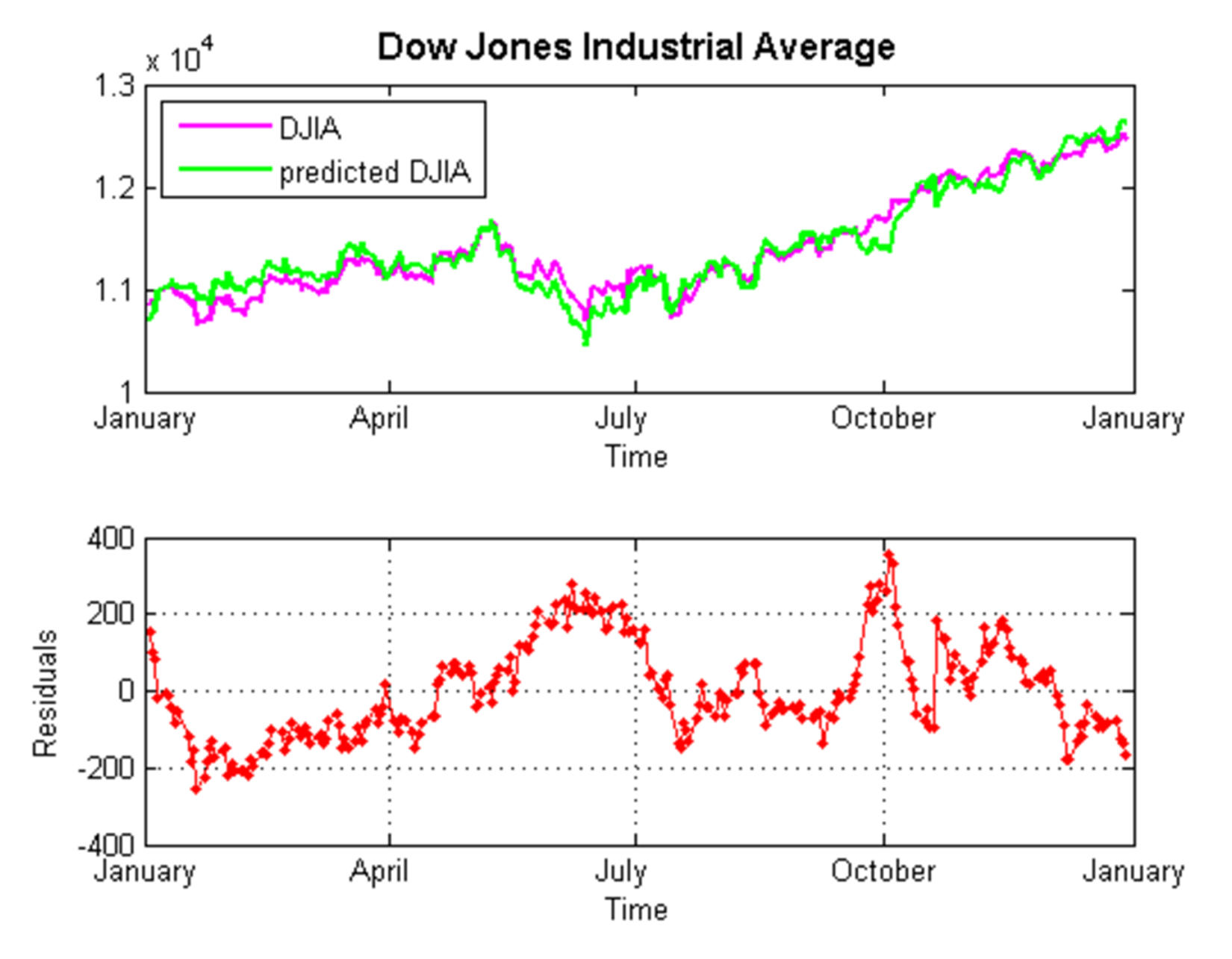Figure 1.20: Step-wise regression example

Neural Network Toolbox

ANNs are a computational model used in computer science, built on a large series of simple neural units, called artificial neurons, which draw inspiration from the behavior observed in the axons of a human brain. Each neural unit is connected with many others, and such link defines the activation status of the adjacent neural units. Every single neural unit performs calculations using the summation function. The models based on ANNs are self-learning and training, rather than explicitly programmed, which his is particularly suitable in cases where the solution function is hard to express in a traditional computer program.

The Neural Network Toolbox provides algorithms, pre-trained models, and apps to create, train, visualize, and simulate neural networks with one hidden layer (called shallow neural network) and neural networks with several hidden layers (called deep neural networks). Through the use of the tools offered, we can perform classification, regression, clustering, dimensionality reduction, time series forecasting, and dynamic system modeling and control.

Deep learning networks include convolutional neural networks (CNNs) and autoencoders for image classification, regression, and feature learning. For training sets of moderated sized, we can quickly apply deep learning by performing transfer learning with pre-trained deep networks. To make working on large amounts of data faster, we can use the Parallel Computing Toolbox (another MATLAB toolbox) to distribute computations and data across multicore processors and GPUs on the desktop, and we can scale up to clusters and clouds with MATLAB Distributed Computing Server.

Here is a descriptive list of the key features of this tool; you will find the main topics of the machine learning field here:

• Deep learning with CNNs (for classification and regression) and autoencoders (for feature learning)
• Transfer learning with pre-trained CNNs models and models from the Caffe model zoo
• Training and inference with CPUs or multi-GPUs on desktops, clusters, and clouds
• Unsupervised learning algorithms, including self-organizing maps and competitive layers

In the following screenshot, the product capabilities of the Neural Networks Toolbox are shown, extracted from the MathWorks site: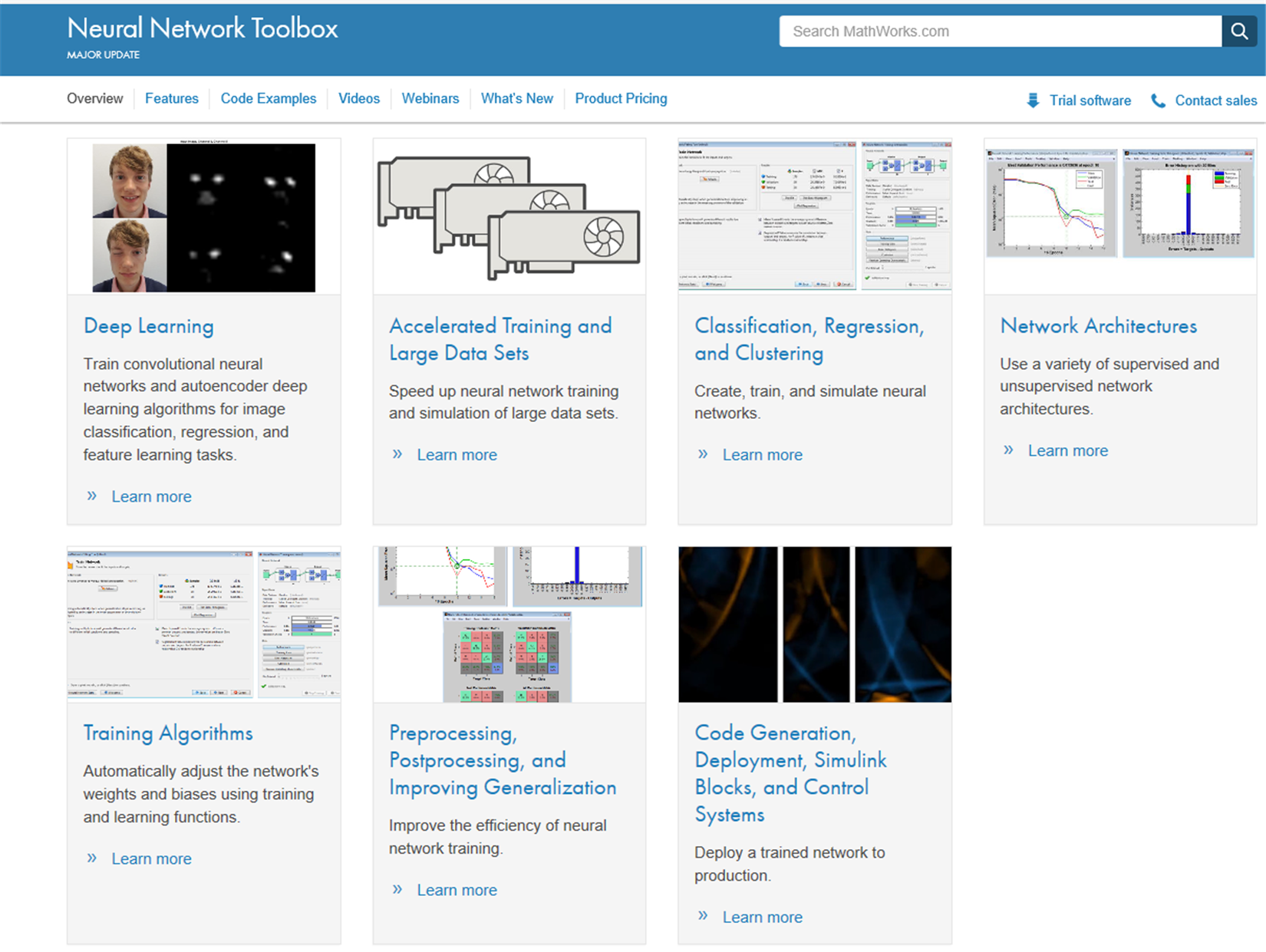Figure 1.21: Product capabilities of the Neural Networks Toolbox

• Supervised learning algorithms, including multilayer, radial basis, Learning Vector Quantization (LVQ), time-delay, nonlinear autoregressive (NARX), and Recurrent Neural Network (RNNs)
• Apps for data fitting, pattern recognition, and clustering
• Preprocessing, postprocessing, and network visualization for improving training efficiency and assessing network performance

Note

For a more comprehensive overview of the Neural Network Toolbox's capabilities, you can connect to the manufacturer's website at the following link:  https://www.mathworks.com/products/ neural-network.html.

Statistics and algebra in MATLAB

Machine learning is an interdisciplinary field; it includes statistics, probability theory, algebra, computer science, and much more. These disciplines come together in algorithms capable of learning iteratively from data and finding hidden insights that can be used to create intelligent applications. In spite of the immense possibilities offered by machine learning, a thorough mathematical understanding of many of these disciplines is necessary for a good understanding of the inner workings of the algorithms and getting good results.

There are many reasons why the statistics and algebra is important to build a machine learning system. Some of them are highlighted as follows:

• Select the right algorithm in terms of accuracy, training time, number of parameters, number of features, and complexity of the model
• Correctly set the parameters and choose validation strategies
• Recognize underfitting and overfitting
• Put appropriate confidence interval and uncertainty

MATLAB offers several functions that allow us to perform statistical analyses and algebraic operations on our data. For example, in MATLAB, computing descriptive statistics from sample data really is a breeze. It is possible to measure central tendency, dispersion, shape, correlation, covariance, quantiles, percentiles and much more. In addition, we can tabulate and cross-tabulate data, and compute summary statistics for grouped data. In case of missing (NaN) values, MATLAB arithmetic operation functions return NaN. To solve this problem, available functions in Statistics and Machine Learning Toolbox ignore these missing values and return a numerical value calculated using the remaining values.

Furthermore we can use statistical visualization to understand how data is distributed and how that compares to other datasets and distributions. In MATLAB, we may explore single-variable distributions using univariate plots such as box plots and histograms. As well as we can discover the relationships between variables applying bivariate plots such as grouped scatter plots and bivariate histograms. We visualize the relationship between multiple variables using multivariate plots such as Andrews and glyph plots. Finally we may customize our plot by adding case names, least-squares lines, and reference curves.

As for statistical analysis, and also for linear algebra, MATLAB offers many solutions ready to use. To remind you, linear algebra is the study of linear equations and their properties and is based on numerical matrices. MATLAB offers many tools for manipulating matrices, easily understood by people who are not experts in them. MATLAB makes it easy to perform computations with vectors and matrices.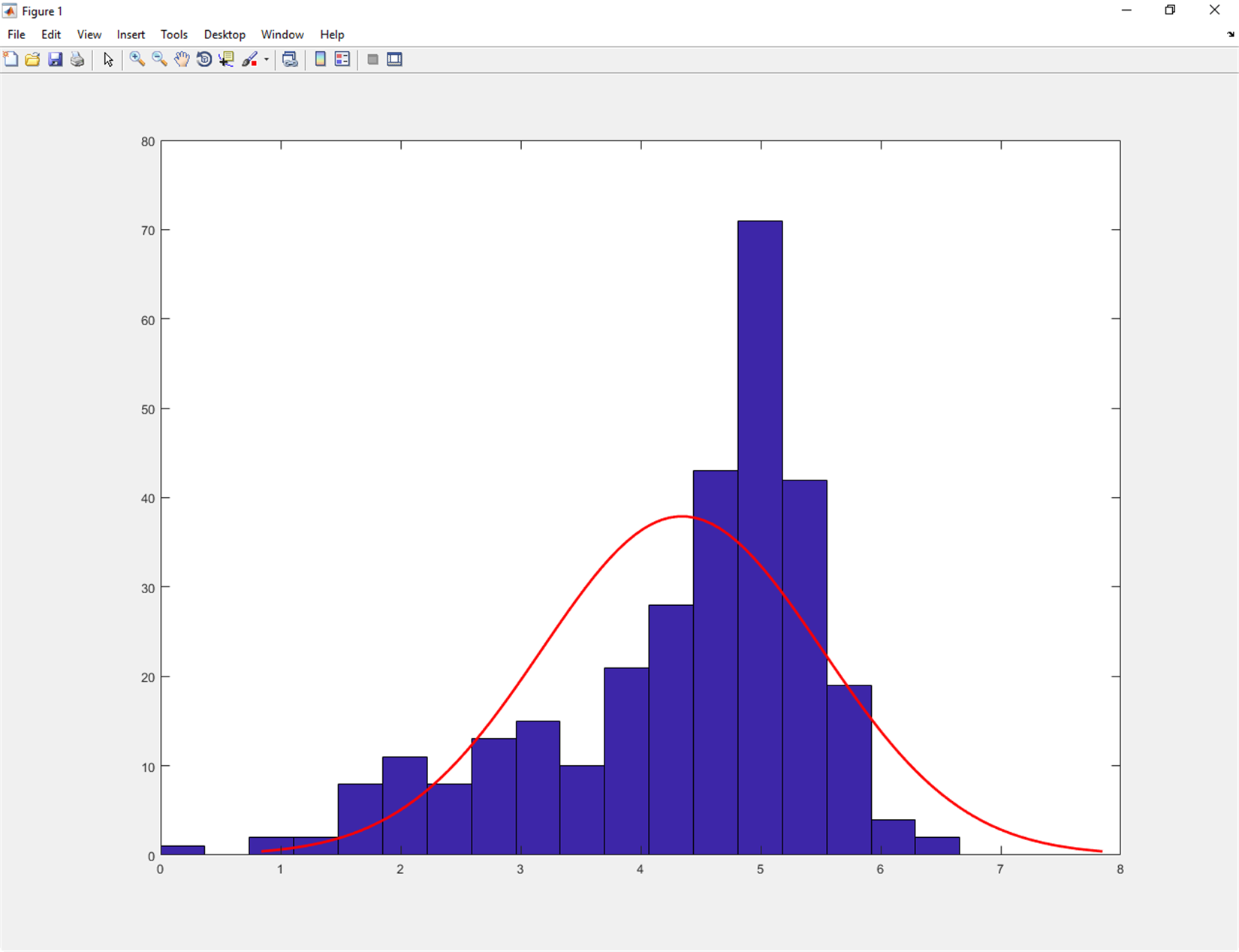Figure 1.22: Histogram of the sample data with a normal density fit

MATLAB provides several functions for:

• Matrix operations and transformations
• Linear equations
• Matrix factorization and decomposition
• Eigenvalues and eigenvectors
• Matrix analysis and vector calculus
• Normal forms and special matrices
• Matrix functions

With the help of these functions, performing linear algebra tasks in a MATLAB environment is really easy.

Summary

In this chapter, we explored the amazing world of machine learning and took a tour of the most popular machine learning algorithms to choose the right one for our needs. To understand what is most suitable for our needs, we learned to perform a preliminary analysis. Then we analyzed how to build machine learning models step by step.

Afterwards, we discovered the machine learning capabilities in MATLAB for classification, regression, clustering, and deep learning, including apps for automated model training and code generation. We verified the MATLAB system requirements and platform availability for a correct installation.

Finally, we introduced the Statistics and Machine Learning Toolbox and Neural Network Toolbox. We learned what we can do with these tools, and what algorithms we need to use to solve our problems. We understood the role of statistics and algebra in machine learning and how MATLAB can help us.

In the next chapter, we will learn how to easily interact with the MATLAB workspace, import and organize our data in MATLAB, export data from the workspace, and organize the data in the correct format for the next phase of data analysis.